Algebra 1 3-4 Guided Practice: Solving Multi-Step Inequalities
starstarstarstarstarstarstarstarstarstar
by Matthew Richardson
| 14 Questions1
1
10
Solve It! Math Club members are selling Pi Day T-shirts for \$7.50 each. The goal is to raise \$500 by Friday. The figure shows how much they have raised by Wednesday. What is the minimum number of shirts they must still sell in order to reach their goal?
19
21
20
222
3
2
10
Take Note: Describe how the process of solving a multi-step inequality is similar to solving a multi-step equation.
3
5
Take Note: What is the impact that multiplying or dividing both sides of a multi-step inequality by a negative has on the inequality symbol?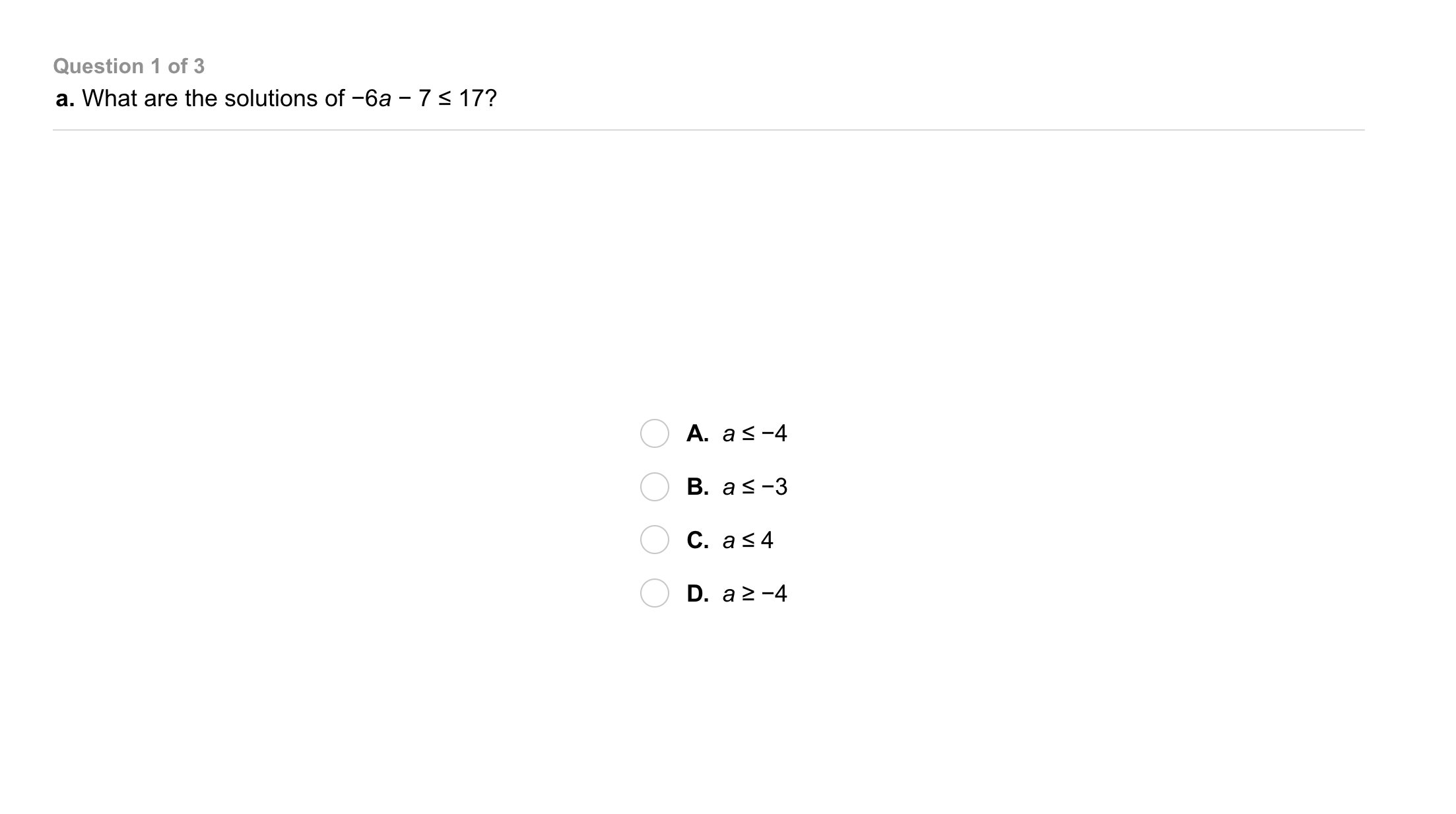4
4
10
Problem 1 Got It?
A
B
C
D5
5
10
Problem 1 Got It?
A
B
C
D6
6
10
Problem 1 Got It?
A
B
C
D7
7
10
Problem 2 Got It?
A
B
C
D8
8
10
Problem 3 Got It?
A
B
C
D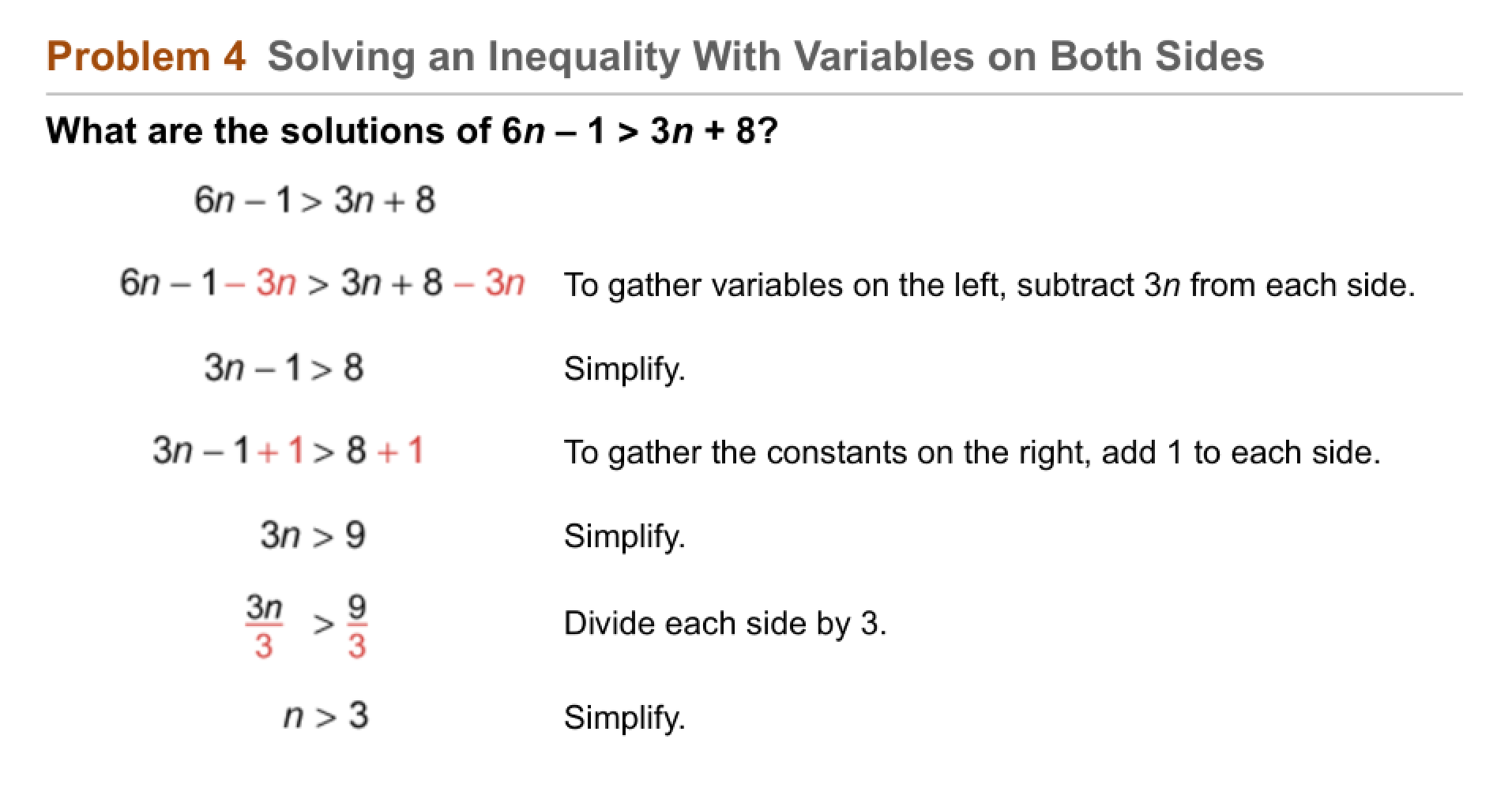9
9
10
Problem 4 Got It? Reasoning: The first step in solving Problem 4 was to subtract 3n from each side of the inequality. What else could have been the first step in solving the inequality? Explain.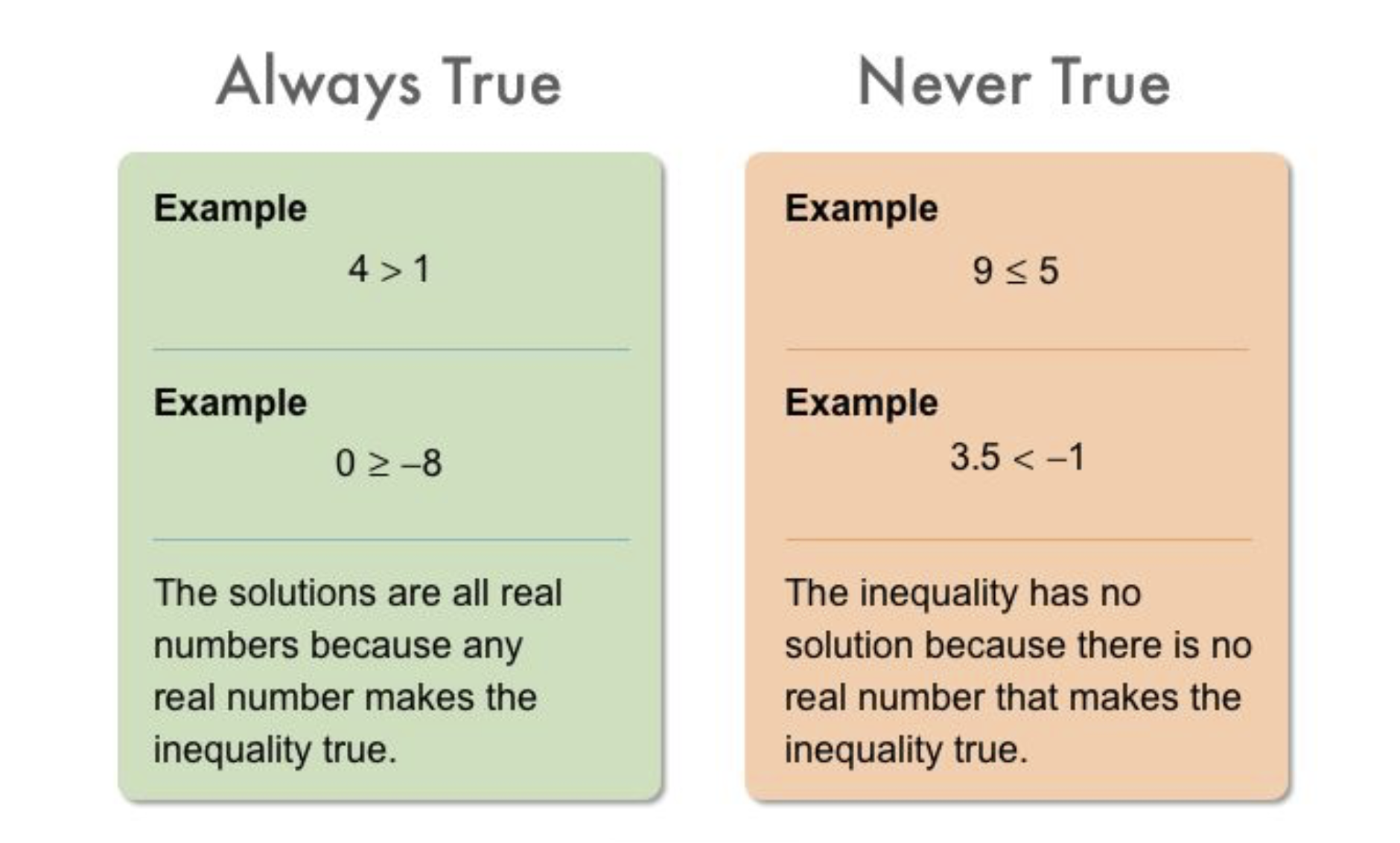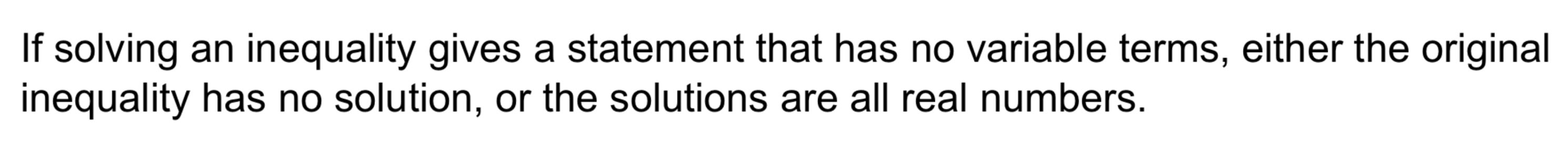10
11
10
10
Take Note: Provide an example of an inequality that is never true.
11
10
Take Note: Provide an example of an inequality that is always true.12
12
10
Problem 5 Got It?
A
B
C
D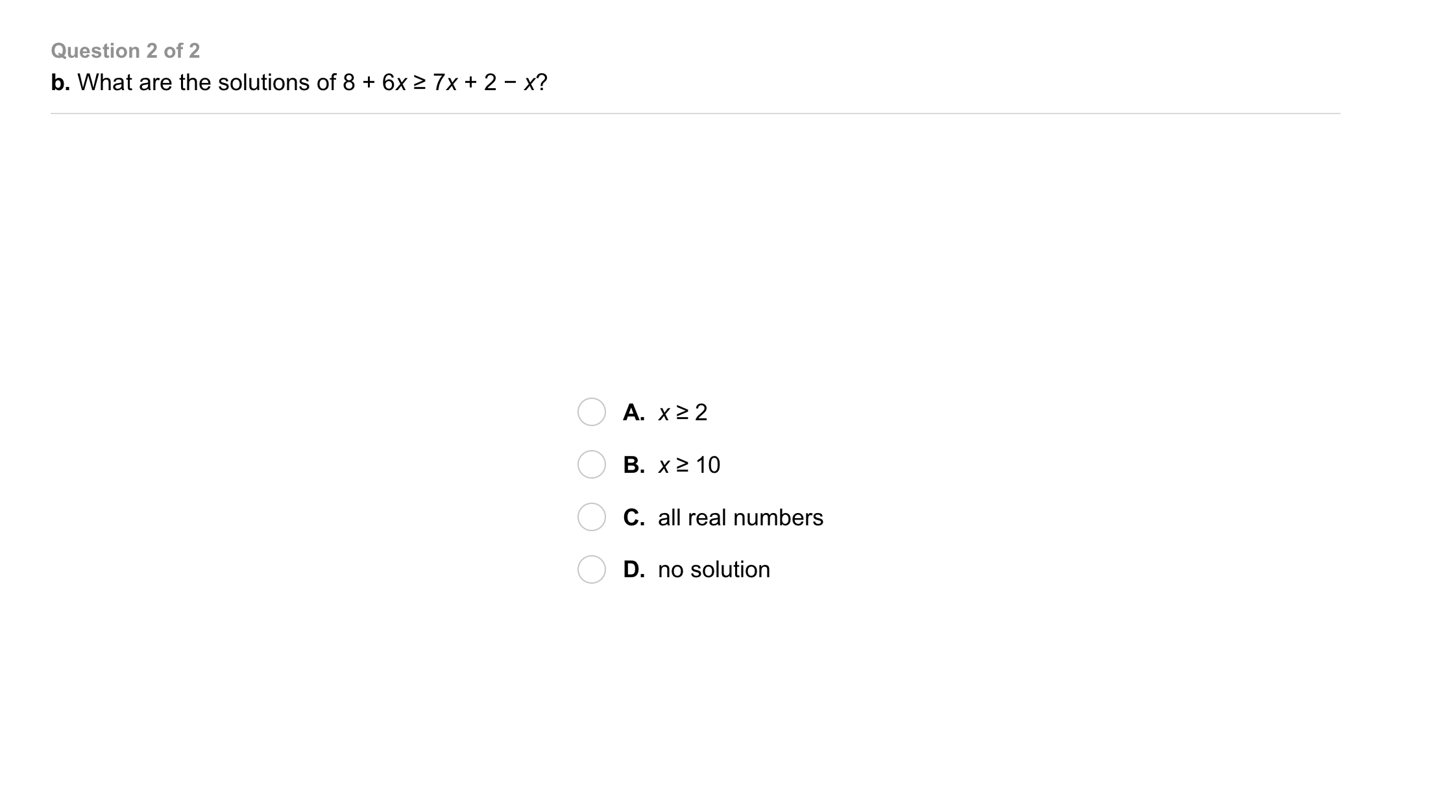13
13
10
Problem 5 Got It?
A
B
C
D14
14
10
Take Note: Summarize the mathematical content of this lesson. What topics, ideas, and vocabulary were introduced?